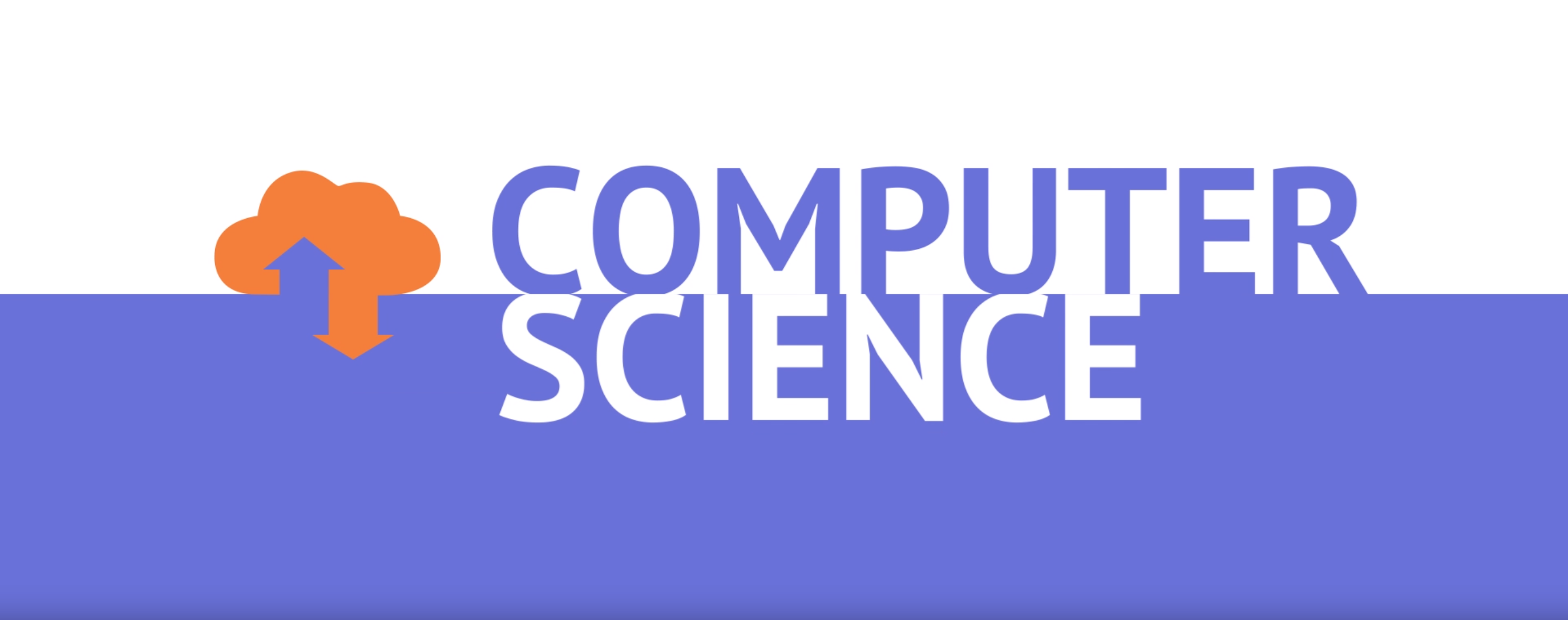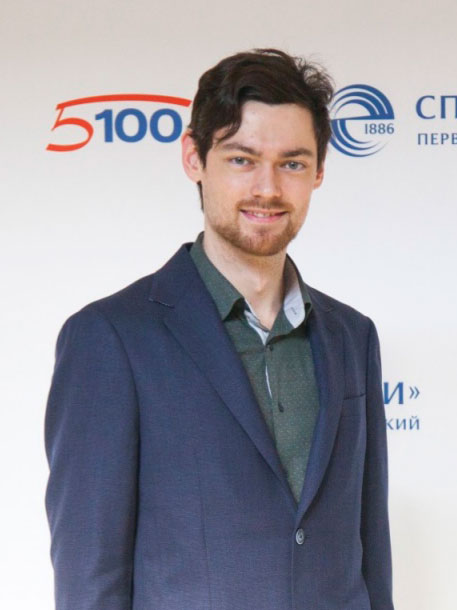# Computer Science

St. Petersburg Electrotechnical University 'LETI'
Enrollment in this course is by invitation onlyThe course is dedicated to studying basics of computer science, both theoretical and applied. The theoretical part of the course includes principles of the computer operation, numeral systems, Boolean algebra, information and forms of its presentation. The practical part of the course includes text editors, spreadsheets, operating systems, programming. This knowledge and skills allows students successful proceeding the bachelor’s program.

The online course is developed for use in distance and blended learning.

## Objectives

• The study of the basics of the information theory, the algebra of logic, number systems, the theory of algorithms, the basic principles of building a computer and the basics of programming.
• Formation of the ability of using computers to solve the most common problems of data processing.

## Common Course Outline

• Module 1. Basic principles of functioning of PC
• Lesson 1.1 Сomputer architecture and classification
• Lesson 1.2 Internal devices of PC
• Lesson 1.3 CPU
• Lesson 1.4 External devices
• Module 2. Numeral systems
• Lesson 2.1 Numeral systems: overview
• Lesson 2.2 Positional numeral systems
• Lesson 2.3 Addition and multiplication in positional numeral systems
• Lesson 2.4 Fractions and division in positional numeral systems
• Lesson 2.5 Conversion between numeral systems
• Lesson 2.6 Binary numeral system and operations in it
• Lesson 2.7 Octal numeral system and operations in it
• Lesson 2.8 Hexadecimal numeral system and operations in it
• Lesson 2.9 Ternary numeral system
• Lesson 2.10 Gray code and its generalization
• Module 3. Boolean Algebra
• Lesson 3.1 Logical elements in computer
• Lesson 3.2 Basic operations
• Lesson 3.3 Secondary operations
• Lesson 3.4 Laws of Boolean algebra
• Lesson 3.5 Propositional logic
• Lesson 3.6 Normal forms
• Lesson 3.7 Simplification of boolean functions
• Lesson 3.8 Minimization of boolean functions
• Lesson 3.9 Karnaugh map
• Lesson 3.10 Bit operations
• Module 4. Information and forms of its presentation
• Lesson 4.1 Information in PC
• Lesson 4.2 Signal and its types
• Lesson 4.3 Quantity of information, by Shannon
• Lesson 4.4 Shannon theorem
• Lesson 4.5 IEEE 754 standard
• Lesson 4.8 Multiplication and division in PC
• Lesson 4.9 Karatsuba algorithm
• Lesson 4.10 ASCII and UNICODE
• Module 5. Text editors
• Lesson 5.1 Overview of text editors
• Lesson 5.2 MS Word: introduction to interface
• Lesson 5.3 Basic text manipulation
• Lesson 5.4 Text and page formatting
• Lesson 5.5 Images and tables
• Lesson 5.6 Special symbols and formulas
• Lesson 5.8 Review
• Lesson 5.9 Styles and templates
• Lesson 5.10 Macros
• Lesson 6.1 Overview of spreadsheet editors
• Lesson 6.2 MS Excel: introduction to interface
• Lesson 6.3 Basic spreadsheet manipulations
• Lesson 6.4 Addresses of cells. Formulas
• Lesson 6.5 Basic mathematical operations
• Lesson 6.6 Diagrams
• Lesson 6.7 Filtering and conditional formatting
• Lesson 6.8 Functions, part 1
• Lesson 6.9 Functions, part 2
• Lesson 6.10 Functions, part 3
• Module 7. PC operating systems
• Lesson 7.1 Function and history of operating systems
• Lesson 7.2 Types of operating systems
• Lesson 7.3 Overview of Windows
• Lesson 7.4 Overview of Linux
• Lesson 7.5 Operating systems for mobile devices
• Module 8. Fundamentals of algorithmization and programming
• Lesson 8.1 Algorithm. Basic ideas
• Lesson 8.2 Basic structures. Flowchart and pseudocode notation
• Lesson 8.3 Python 3.0. Variables. Input and output
• Lesson 8.4 Conditionals
• Lesson 8.5 Data types
• Lesson 8.6 Mathematical operations
• Lesson 8.7 Loops
• Lesson 8.8 Arrays and lists
• Lesson 8.9 Strings
• Lesson 8.10 Functions and modules
• Lesson 8.11 Classes
• Lesson 8.12 NymPy library
• Lesson 8.13 Matplotlib library

For the attestation, the student should:

• pass entry quiz (10% of the final grade);
• pass control tests for the course modules (60% of the final grade);
• pass examination testing (20% of the final grade).

## Rating system

The results of tests and completed assignments are evaluated according to the rating system, the total amount of accrued interest for all types of activities is converted into an assessment on a four-point scale:

• «excellent» – at least 90% of successfully performed;
• «good» – at least 70%, less than 90%;
• «satisfactory» – at least 60%, less than 70%;
• «unsatisfactory» – less than 60%.

## Entry requirements and target audience

The course is designed for school students of higher grades and bachelors of the 1st year of study. It can be used to train masters and specialists in the engineering and humanitarian sciences fields not related to computer science.

If you pass an introduction test for this course you will be accepted on a course.

## Technical requirements

Review the technical requirements to access the course and complete it successfully.

## Course authors### Karimov Arthur Iskandarovich

PhD, Associate professor of Computer-Aided Design Department

St.-Petersburg Electrotechnical University «LETI»
1. Course Number

CS101
2. Classes Start

3. Classes End

4. Estimated Effort

4 hours per week
5. Number of Weeks

12